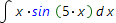# Online indefinite integral calculator

Indefinite integral of the given function f(x) is called the set of all its antiderivatives:

f(x) dx=F(x) +Const

In order to calculate indefinite integral of the given function, one need to use integral table and integration rules or use our free online calculator.

Calculator is able to find step by step solution for many types of the integrals. The solution received, contains description of the every step.

All advantages of our step by step solution can be revealed here. The step by step solution example can be found here.

## Choose expression input type:

Expression input type: